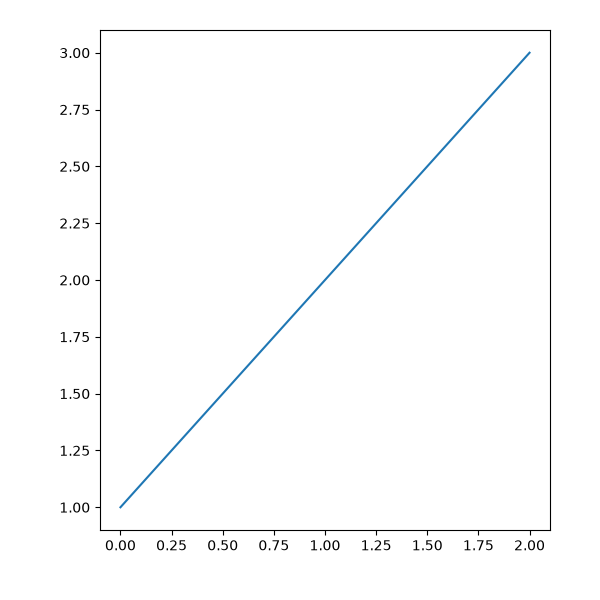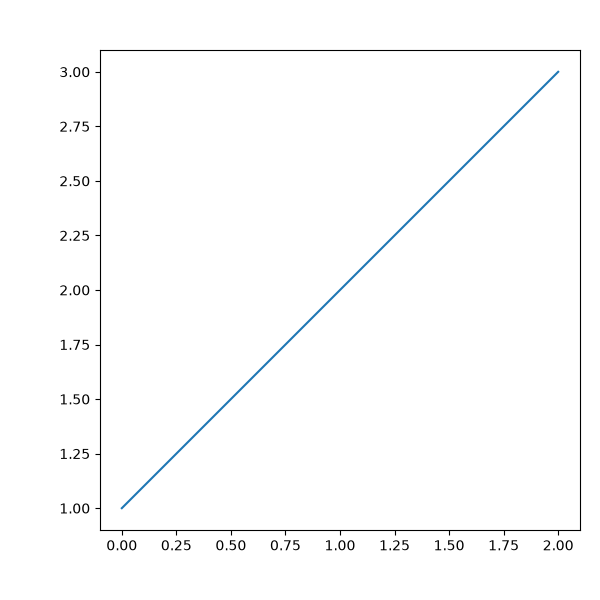# Axes with a fixed physical size#

Note that this can be accomplished with the main library for Axes on Figures that do not change size: Adding single Axes at a time

import matplotlib.pyplot as plt

from mpl_toolkits.axes_grid1 import Divider, Size

fig = plt.figure(figsize=(6, 6))

# The first items are for padding and the second items are for the axes.
# sizes are in inch.
h = [Size.Fixed(1.0), Size.Fixed(4.5)]
v = [Size.Fixed(0.7), Size.Fixed(5.)]

divider = Divider(fig, (0, 0, 1, 1), h, v, aspect=False)
# The width and height of the rectangle are ignored.

axes_locator=divider.new_locator(nx=1, ny=1))

ax.plot([1, 2, 3])fig = plt.figure(figsize=(6, 6))

# The first & third items are for padding and the second items are for the
# axes. Sizes are in inches.
h = [Size.Fixed(1.0), Size.Scaled(1.), Size.Fixed(.2)]
v = [Size.Fixed(0.7), Size.Scaled(1.), Size.Fixed(.5)]

divider = Divider(fig, (0, 0, 1, 1), h, v, aspect=False)
# The width and height of the rectangle are ignored.